Questions - Forming equations and solving

Chapter 12 Class 12 Linear Programming
Serial order wise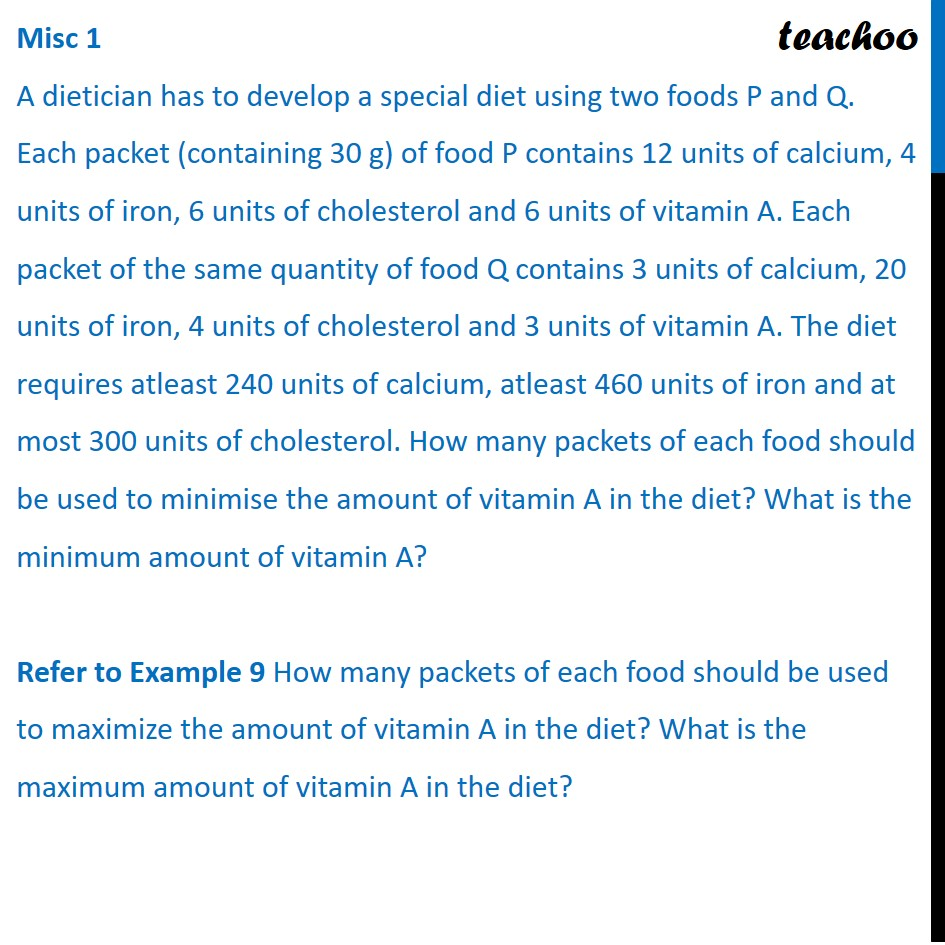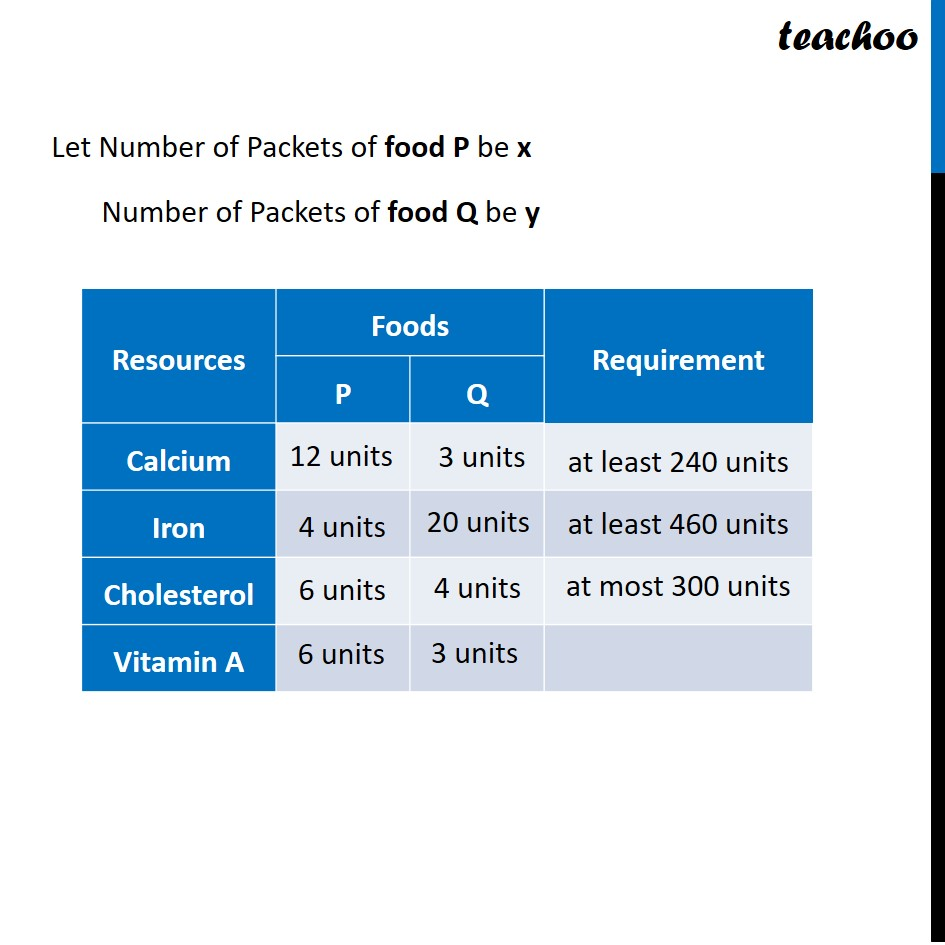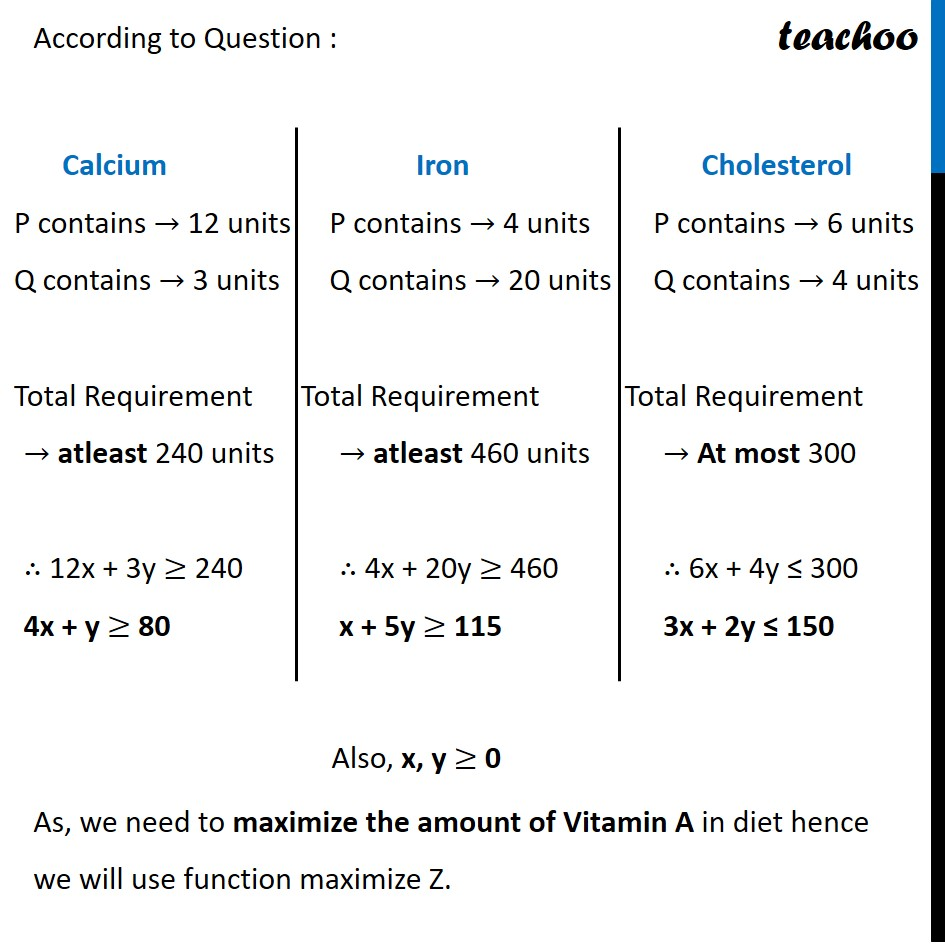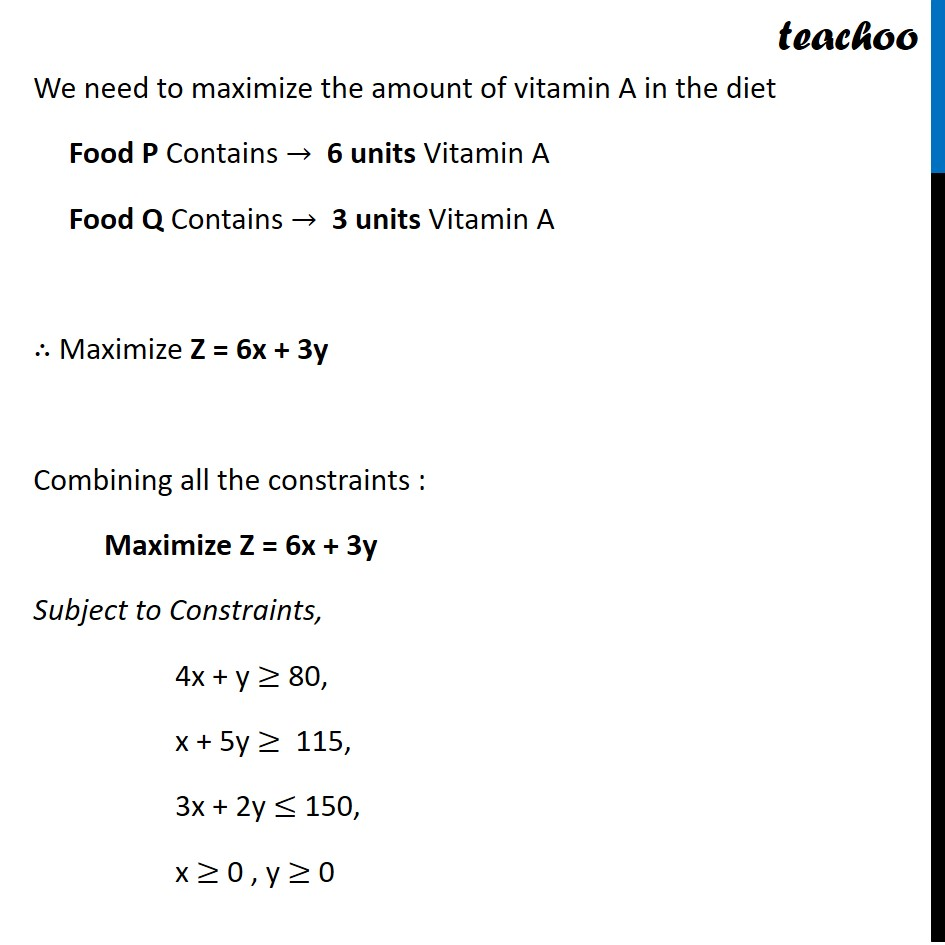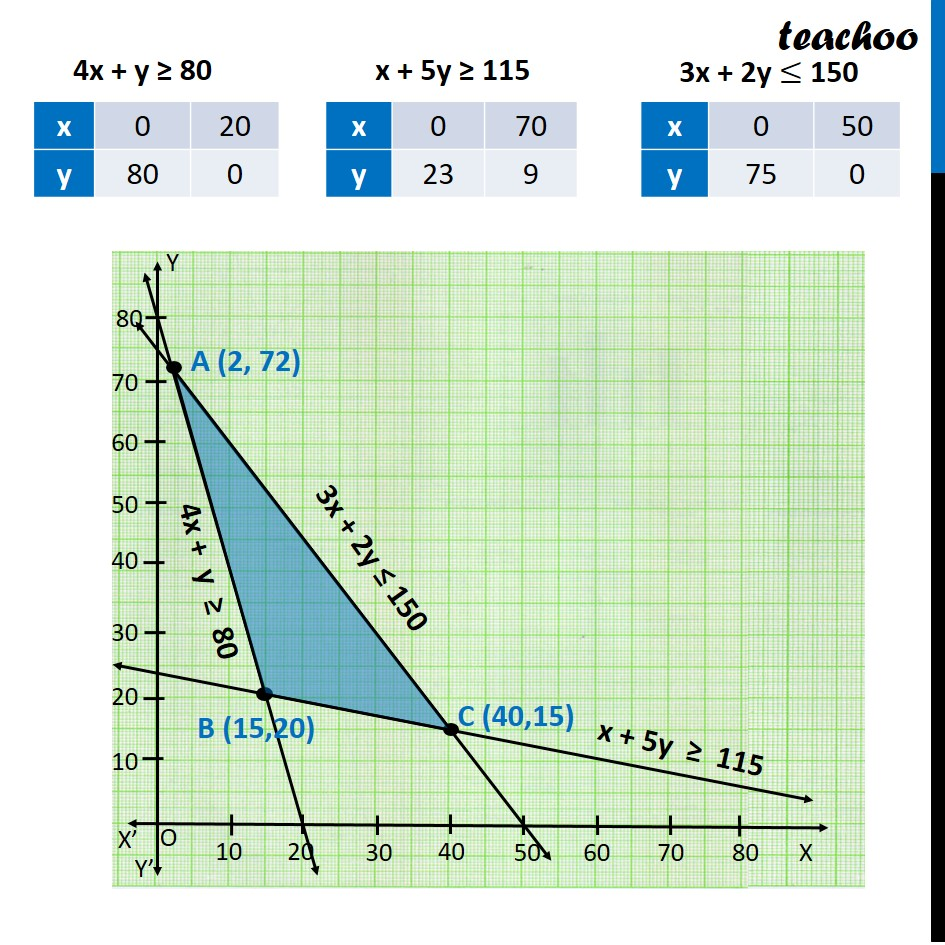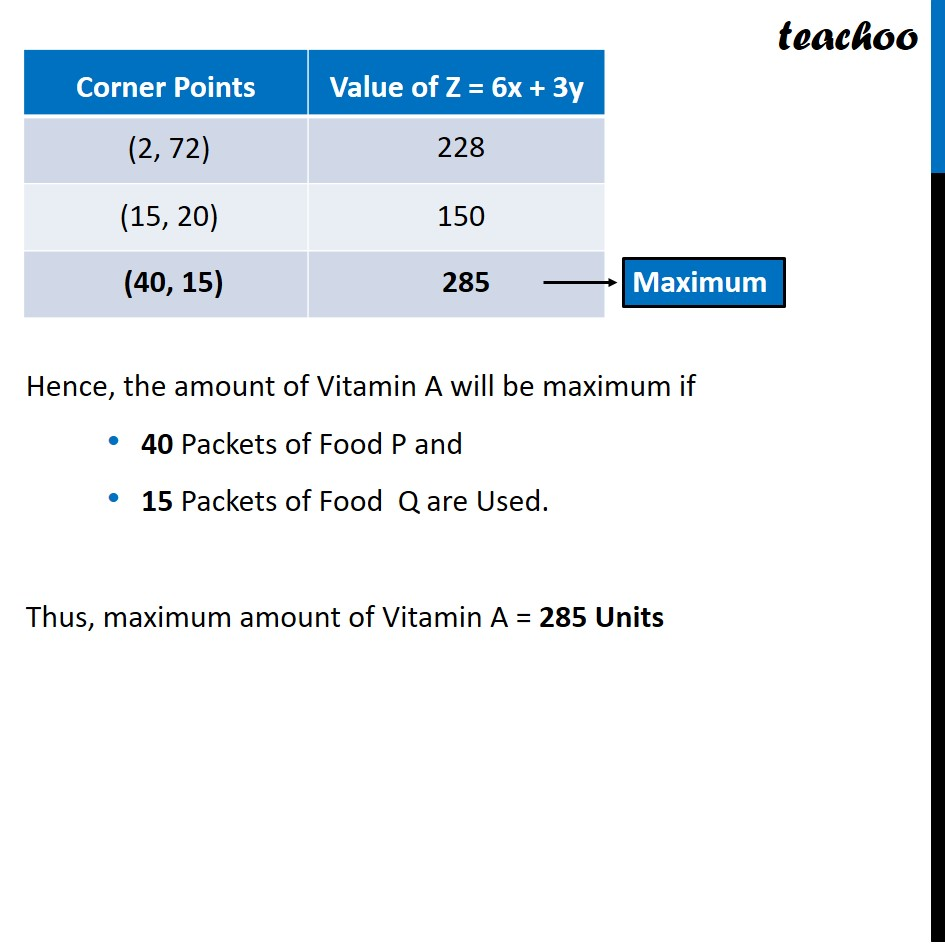Learn in your speed, with individual attention - Teachoo Maths 1-on-1 Class

### Transcript

Misc 1 A dietician has to develop a special diet using two foods P and Q. Each packet (containing 30 g) of food P contains 12 units of calcium, 4 units of iron, 6 units of cholesterol and 6 units of vitamin A. Each packet of the same quantity of food Q contains 3 units of calcium, 20 units of iron, 4 units of cholesterol and 3 units of vitamin A. The diet requires atleast 240 units of calcium, atleast 460 units of iron and at most 300 units of cholesterol. How many packets of each food should be used to minimise the amount of vitamin A in the diet? What is the minimum amount of vitamin A? Refer to Example 9 How many packets of each food should be used to maximize the amount of vitamin A in the diet? What is the maximum amount of vitamin A in the diet? Let Number of Packets of food P be x Number of Packets of food Q be y According to Question : As, we need to maximize the amount of Vitamin A in diet hence we will use function maximize Z. Calcium P contains → 12 units Q contains → 3 units Total Requirement → atleast 240 units ∴ 12x + 3y ≥ 240 4x + y ≥ 80 Iron P contains → 4 units Q contains → 20 units Total Requirement → atleast 460 units ∴ 4x + 20y ≥ 460 x + 5y ≥ 115 Cholesterol P contains → 6 units Q contains → 4 units Total Requirement → At most 300 ∴ 6x + 4y ≤ 300 3x + 2y "≤" 150 We need to maximize the amount of vitamin A in the diet Food P Contains → 6 units Vitamin A Food Q Contains → 3 units Vitamin A ∴ Maximize Z = 6x + 3y Combining all the constraints : Maximize Z = 6x + 3y Subject to Constraints, 4x + y ≥ 80, x + 5y ≥ 115, 3x + 2y ≤ 150, x ≥ 0 , y ≥ 0 4x + y ≥ 80 x + 5y ≥ 115 3x + 2y ≤ 150 Hence, the amount of Vitamin A will be maximum if 40 Packets of Food P and 15 Packets of Food Q are Used. Thus, maximum amount of Vitamin A = 285 Units